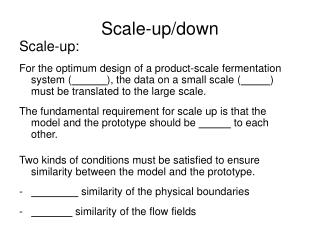# Scale-up/down - PowerPoint PPT PresentationDownload PresentationScale-up/down

Scale-up/downDownload Presentation## Scale-up/down

- - - - - - - - - - - - - - - - - - - - - - - - - - - E N D - - - - - - - - - - - - - - - - - - - - - - - - - - -
##### Presentation Transcript

1. Scale-up/down Scale-up: For the optimum design of a product-scale fermentation system (), the data on a small scale () must be translated to the large scale. The fundamental requirement for scale up is that the model and the prototype should be to each other. Two kinds of conditions must be satisfied to ensure similarity between the model and the prototype. • similarity of the physical boundaries - similarity of the flow fields

2. Scale-up/down Scale-up: • Geometric similarity of the physical boundaries: - of reactor - all linear dimensions of the model must be related to the corresponding dimensions of the prototype by o . i.e. Keep the ratio of the height H to diameter Dt (tank) same in the model and prototype. Normally H/Dt is 2~3. Scale-up factor: Ratio of surface to volume decreases during scale-up.

3. Example: Scale-up 2 litre cylindrical tank (Vm) is scaled up to 2000 litre (Vp) fermenter by geometrical similarity, H/Dt=2, impeller diameter Di,m =3.24 cm, what are the dimensions of the model (Hm, Dt,m), and prototype (Hp, Dt,p, Di,p)?

4. Scale-up: • Dynamic similarity of the flow fields To achieve dynamic similarity in a stirred-tank reactor, scale-up can be based on the following criteria in addition to geometric similar boundaries. constant power input per volume: . or constant liquid circulation rate inside the reactor: . (pumping rate of impeller per unit volume) constant impeller tip speed (shear): . constant Renolds number: . V: working volume P0: energy input (W); N: impeller speed (rpm); ρ: density (kg/m3) Di: impeller diameter (m),30-40% of the diameter of the tank (Dt) µ: viscosity (kg/ms); g: acceleration due to gravity (m/s2).

5. Scale-up: Relating the above criteria to impeller diameter Di and speed N: (Perry’s Chemical Engineer’s Handbook, 7th Ed. Page.18-11)

6. Scale-up/down Scale-up: In scale-up of a stirred-tank reactor, the design calculations are as follows: • Determine the . • Based on the geometric similarity, calculate the dimensions of the prototype (height H and diameter Dt of tanks, impeller diameter Di) by multiplying that of the model. . • Select criterion related to properties and keep it constant in both the model and the prototype. • Determine the parameters such as or diameter for the scale-up reactor.

7. Scale-up For an example, 2 litre vessel (Vm) is scaled up to 2000 litre (Vp) fermenter, (H/Dt)model=2, impeller diameter Di,m is 3.24 cm, impeller speed Nm is 500 rpm, what is the impeller speed of the larger reactor for - constant impeller tip speed - constant Renolds number

8. Scale-up/down Scale-down: To provide an experimental system at a smaller scale that duplicates the environment that exists at the larger scale. • Mimic the production facilities at a smaller scale • Parameters can be tested more quickly and inexpensively than at the production scale. Design calculations used in scale-down are the same as that in scale-up. Please read the example 10.3